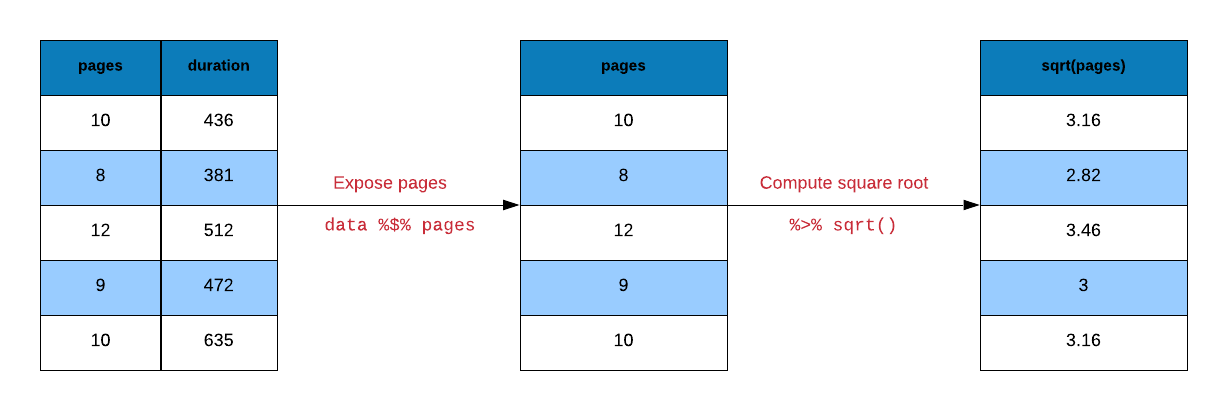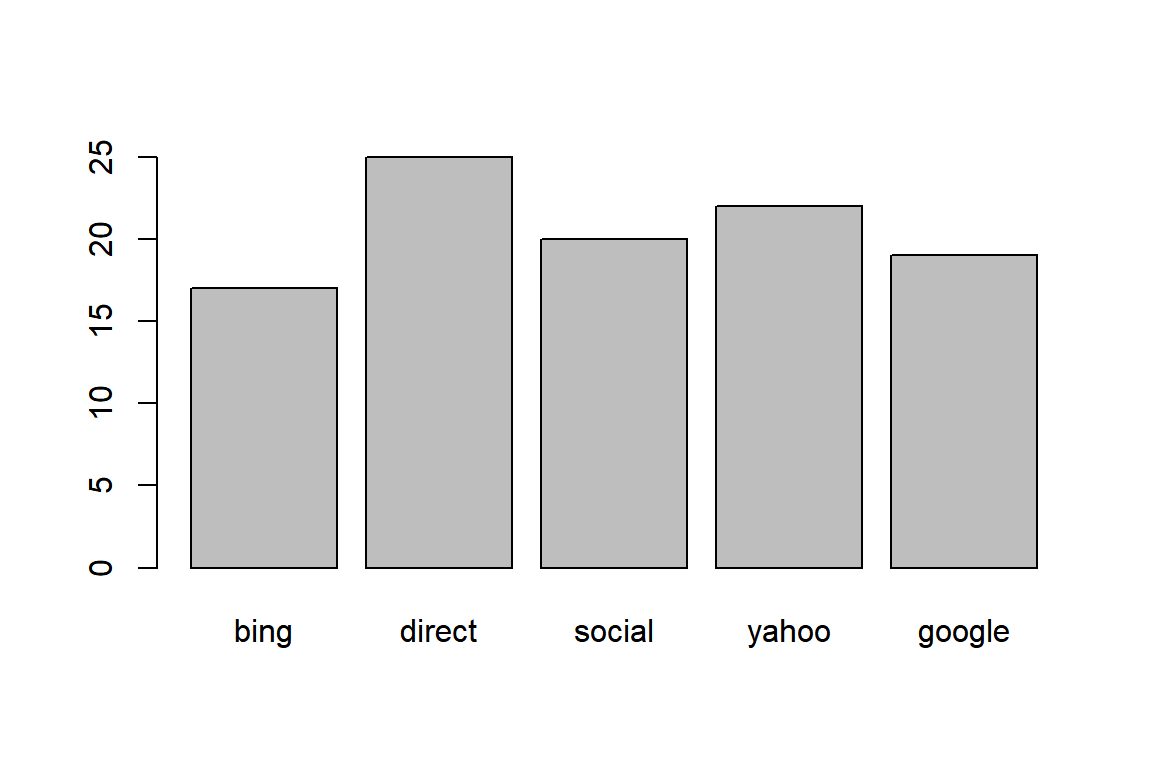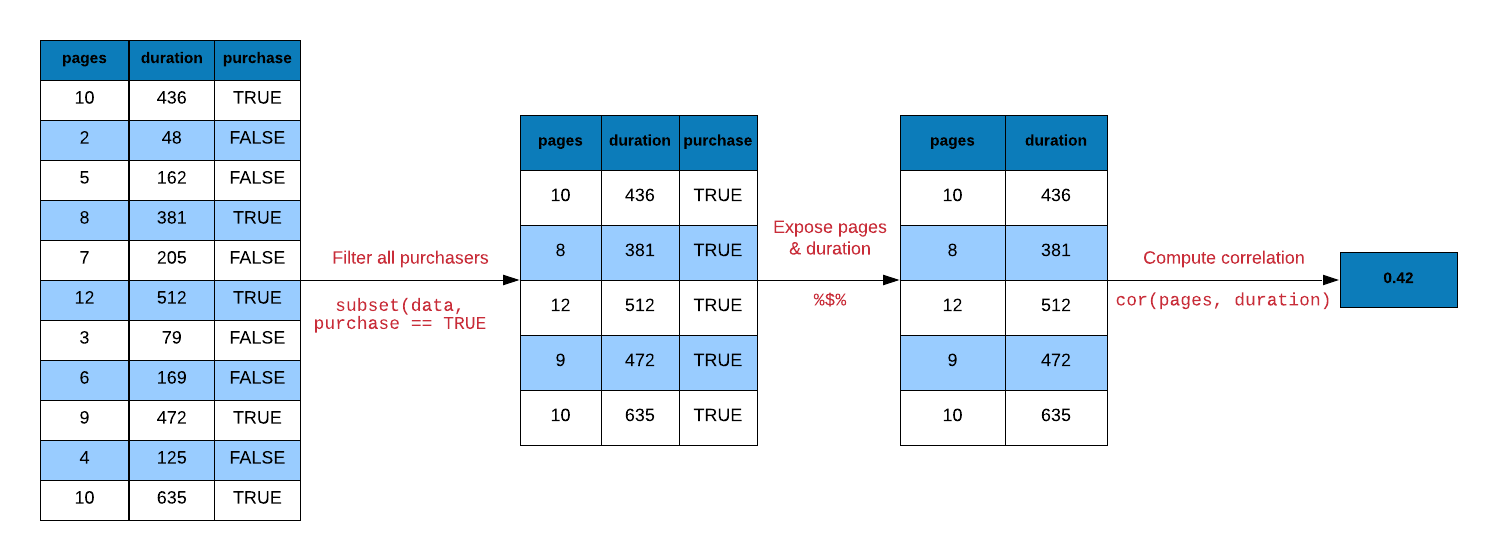October 9, 2018
By

Want to share your content on R-bloggers? click here if you have a blog, or here if you don't.

## Introduction

R code contain a lot of parentheses in case of a sequence of multiple operations. When you are dealing with
complex code, it results in nested function calls which are hard to read and maintain. The magrittr package by Stefan Milton Bache provides pipes enabling us to write R code that is readable.

Pipes allow us to clearly express a sequence of multiple operations by:

• structuring operations from left to right
• avoiding
• nested function calls
• intermediate steps
• overwriting of original data
• minimizing creation of local variables

## Pipes

If you are using tidyverse, magrittr will be automatically loaded. We will look at 3 different types of pipes:

• `%>%` : pipe a value forward into an expression or function call
• `%<>%`: result assigned to left hand side object instead of returning it
• `%\$%` : expose names within left hand side objects to right hand side expressions

## Libraries, Code & Data

We will use the following packages in this post:

You can find the data sets here and the codes
here.

``````library(magrittr)
library(dplyr)
library(stringr)
library(purrr)``````

## Data

``````ecom <-
col_types = cols_only(
referrer = col_factor(levels = c("bing", "direct", "social", "yahoo", "google")),
n_pages = col_double(), duration = col_double(), purchase = col_logical()
)
)

ecom``````
``````## # A tibble: 1,000 x 4
##    referrer n_pages duration purchase
##
##  1 google         1      693 FALSE
##  2 yahoo          1      459 FALSE
##  3 direct         1      996 FALSE
##  4 bing          18      468 TRUE
##  5 yahoo          1      955 FALSE
##  6 yahoo          5      135 FALSE
##  7 yahoo          1       75 FALSE
##  8 direct         1      908 FALSE
##  9 bing          19      209 FALSE
## 10 google         1      208 FALSE
## # ... with 990 more rows``````

We will create a smaller data set from the above data to be used in some examples:

``````ecom_mini <- sample_n(ecom, size = 10)
ecom_mini``````
``````## # A tibble: 10 x 4
##    referrer n_pages duration purchase
##
##  1 google         2       54 FALSE
##  2 direct        15      330 FALSE
##  3 yahoo          1       47 FALSE
##  4 yahoo          1      668 FALSE
##  5 google        10      180 FALSE
##  6 social         2       56 FALSE
##  7 direct         1      675 FALSE
##  8 google         1      523 FALSE
##  9 bing           1      999 FALSE
## 10 bing           8      120 FALSE``````

#### Data Dictionary

• referrer: referrer website/search engine
• n_pages: number of pages visited
• duration: time spent on the website (in seconds)
• purchase: whether visitor purchased

## First Example

Let us start with a simple example. You must be aware of `head()`. If not,
do not worry. It returns the first few observations/rows of data. We can
specify the number of observations it should return as well. Let us use
it to view the first 10 rows of our data set.

``head(ecom, 10)``
``````## # A tibble: 10 x 4
##    referrer n_pages duration purchase
##
##  1 google         1      693 FALSE
##  2 yahoo          1      459 FALSE
##  3 direct         1      996 FALSE
##  4 bing          18      468 TRUE
##  5 yahoo          1      955 FALSE
##  6 yahoo          5      135 FALSE
##  7 yahoo          1       75 FALSE
##  8 direct         1      908 FALSE
##  9 bing          19      209 FALSE
## 10 google         1      208 FALSE``````

#### Using Pipe

Now let us do the same but with `%>%`.

``ecom %>% head(10)``
``````## # A tibble: 10 x 4
##    referrer n_pages duration purchase
##
##  1 google         1      693 FALSE
##  2 yahoo          1      459 FALSE
##  3 direct         1      996 FALSE
##  4 bing          18      468 TRUE
##  5 yahoo          1      955 FALSE
##  6 yahoo          5      135 FALSE
##  7 yahoo          1       75 FALSE
##  8 direct         1      908 FALSE
##  9 bing          19      209 FALSE
## 10 google         1      208 FALSE``````

## Square Root

Time to try a slightly more challenging example. We want the square root of
`n_pages` column from the data set.

``y <- sqrt(ecom_mini\$n_pages)``

Let us break down the above computation into small steps:

• select/expose the `n_pages` column from `ecom` data
• compute the square root
• assign the first few observations to `y`Let us reproduce `y` using pipes.

``````# select n_pages variable and assign it to y
y <-
ecom_mini %\$%
n_pages

# compute square root of y and assign it to y
y %<>% sqrt``````

Another way to compute the square root of y is shown below.

``````y <-
ecom_mini %\$%
n_pages %>%
sqrt()``````

## Visualization

Let us look at a data visualization example. We will create a bar plot to
visualize the frequency of different referrer types that drove purchasers
to the website. Let us look at the steps involved in creating the bar plot:

• extract rows where purchase is TRUE
• select/expose `referrer` column
• tabulate referrer data using `table()`
• use the tabulated data to create bar plot using `barplot()`
``barplot(table(subset(ecom, purchase)\$referrer))``#### Using pipe``````ecom %>%
subset(purchase) %>%
extract('referrer') %>%
table() %>%
barplot()``````## Correlation

Correlation is a statistical measure that indicates the extent to which two or more variables
fluctuate together. In R, correlation is computed using `cor()`. Let us look at the
correlation between the number of pages browsed and time spent on the site for
visitors who purchased some product. Below are the steps for computing correlation:

• extract rows where purchase is TRUE
• select/expose `n_pages` and `duration` columns
• use `cor()` to compute the correlation``````# without pipe
ecom1 <- subset(ecom, purchase)
cor(ecom1\$n_pages, ecom1\$duration)``````
``##  0.4290905``
``````# with pipe
ecom %>%
subset(purchase) %\$%
cor(n_pages, duration)``````
``##  0.4290905``
``````# with pipe
ecom %>%
filter(purchase) %\$%
cor(n_pages, duration)``````
``##  0.4290905``

## Regression

Let us look at a regression example. We regress time spent on the site on
number of pages visited. Below are the steps involved in running the regression:

• use `duration` and `n_pages` columns from ecom data
• pass the above data to `lm()`
• pass the output from `lm()` to `summary()`
``summary(lm(duration ~ n_pages, data = ecom))``
``````##
## Call:
## lm(formula = duration ~ n_pages, data = ecom)
##
## Residuals:
##     Min      1Q  Median      3Q     Max
## -386.45 -213.03  -38.93  179.31  602.55
##
## Coefficients:
##             Estimate Std. Error t value Pr(>|t|)
## (Intercept)  404.803     11.323  35.750  < 2e-16 ***
## n_pages       -8.355      1.296  -6.449 1.76e-10 ***
## ---
## Signif. codes:  0 '***' 0.001 '**' 0.01 '*' 0.05 '.' 0.1 ' ' 1
##
## Residual standard error: 263.3 on 998 degrees of freedom
## Multiple R-squared:   0.04,  Adjusted R-squared:  0.03904
## F-statistic: 41.58 on 1 and 998 DF,  p-value: 1.756e-10``````

#### Using pipe

``````ecom %\$%
lm(duration ~ n_pages) %>%
summary()``````
``````##
## Call:
## lm(formula = duration ~ n_pages)
##
## Residuals:
##     Min      1Q  Median      3Q     Max
## -386.45 -213.03  -38.93  179.31  602.55
##
## Coefficients:
##             Estimate Std. Error t value Pr(>|t|)
## (Intercept)  404.803     11.323  35.750  < 2e-16 ***
## n_pages       -8.355      1.296  -6.449 1.76e-10 ***
## ---
## Signif. codes:  0 '***' 0.001 '**' 0.01 '*' 0.05 '.' 0.1 ' ' 1
##
## Residual standard error: 263.3 on 998 degrees of freedom
## Multiple R-squared:   0.04,  Adjusted R-squared:  0.03904
## F-statistic: 41.58 on 1 and 998 DF,  p-value: 1.756e-10``````

## String Manipulation

We want to extract the first name (jovial) from the below email id and
convert it to upper case. Below are the steps to achieve this:

• split the email id using the pattern `@` using `str_split()`
• extract the first element from the resulting list using `extract2()`
• extract the first element from the character vector using `extract()`
• extract the first six characters using `str_sub()`
• convert to upper case using `str_to_upper()```````email <- '[email protected]'

# without pipe
str_to_upper(str_sub(str_split(email, '@')[], start = 1, end = 6))``````
``##  "JOVIAL"``
``````# with pipe
email %>%
str_split(pattern = '@') %>%
extract2(1) %>%
extract(1) %>%
str_sub(start = 1, end = 6) %>%
str_to_upper()``````
``##  "JOVIAL"``

Another method that uses `map_chr()` from the purrr package.

``````email %>%
str_split(pattern = '@') %>%
map_chr(1) %>%
str_sub(start = 1, end = 6) %>%
str_to_upper()``````
``##  "JOVIAL"``

## Data Extraction

Let us turn our attention towards data extraction. magrittr provides
alternatives to `\$`, `[` and `[[`.

• `extract()`
• `extract2()`
• `use_series()`

#### Extract Column By Name

To extract a specific column using the column name, we mention the name
of the column in single/double quotes within `[` or `[[`. In case of `\$`,
we do not use quotes.

``````# base
ecom_mini['n_pages']``````
``````## # A tibble: 10 x 1
##    n_pages
##
##  1       2
##  2      15
##  3       1
##  4       1
##  5      10
##  6       2
##  7       1
##  8       1
##  9       1
## 10       8``````
``````# magrittr
extract(ecom_mini, 'n_pages') ``````
``````## # A tibble: 10 x 1
##    n_pages
##
##  1       2
##  2      15
##  3       1
##  4       1
##  5      10
##  6       2
##  7       1
##  8       1
##  9       1
## 10       8``````

#### Extract Column By Position

We can extract columns using their index position. Keep in mind that index
position starts from 1 in R. In the below example, we show how to
extract `n_pages` column but instead of using the column name, we use the
column position.

``````# base
ecom_mini``````
``````## # A tibble: 10 x 1
##    n_pages
##
##  1       2
##  2      15
##  3       1
##  4       1
##  5      10
##  6       2
##  7       1
##  8       1
##  9       1
## 10       8``````
``````# magrittr
extract(ecom_mini, 2) ``````
``````## # A tibble: 10 x 1
##    n_pages
##
##  1       2
##  2      15
##  3       1
##  4       1
##  5      10
##  6       2
##  7       1
##  8       1
##  9       1
## 10       8``````

#### Extract Column (as vector)

One important differentiator between `[` and `[[` is that `[[` will
return a atomic vector and not a `data.frame`. `\$` will also return
a atomic vector. In magrittr, we can use `use_series()` in place of
`\$`.

``````# base
ecom_mini\$n_pages``````
``##    2 15  1  1 10  2  1  1  1  8``
``````# magrittr
use_series(ecom_mini, 'n_pages') ``````
``##    2 15  1  1 10  2  1  1  1  8``

#### Extract List Element By Name

Let us convert `ecom_mini` into a list using as.list() as shown below:

``ecom_list <- as.list(ecom_mini)``

To extract elements of a list, we can use `extract2()`. It is an
alternative for `[[`.

``````# base
ecom_list[['n_pages']]``````
``##    2 15  1  1 10  2  1  1  1  8``
``````# magrittr
extract2(ecom_list, 'n_pages')``````
``##    2 15  1  1 10  2  1  1  1  8``

#### Extract List Element By Position

``````# base
ecom_list[]``````
``````##   google direct yahoo  yahoo  google social direct google bing   bing
## Levels: bing direct social yahoo google``````
``````# magrittr
extract2(ecom_list, 1)``````
``````##   google direct yahoo  yahoo  google social direct google bing   bing
## Levels: bing direct social yahoo google``````

#### Extract List Element

We can extract the elements of a list using `use_series()` as well.

``````# base
ecom_list\$n_pages``````
``##    2 15  1  1 10  2  1  1  1  8``
``````# magrittr
use_series(ecom_list, n_pages)``````
``##    2 15  1  1 10  2  1  1  1  8``

## Arithmetic Operations

magrittr offer alternatives for arithemtic operations as well. We will look at
a few examples below.

• `add()`
• `subtract()`
• `multiply_by()`
• `multiply_by_matrix()`
• `divide_by()`
• `divide_by_int()`
• `mod()`
• `raise_to_power()`

``1:10 + 1``
``##    2  3  4  5  6  7  8  9 10 11``
``add(1:10, 1)``
``##    2  3  4  5  6  7  8  9 10 11``
```+`(1:10, 1)``
``##    2  3  4  5  6  7  8  9 10 11``

#### Multiplication

``1:10 * 3``
``##    3  6  9 12 15 18 21 24 27 30``
``multiply_by(1:10, 3)``
``##    3  6  9 12 15 18 21 24 27 30``
```*`(1:10, 3)``
``##    3  6  9 12 15 18 21 24 27 30``

#### Division

``1:10 / 2``
``##   0.5 1.0 1.5 2.0 2.5 3.0 3.5 4.0 4.5 5.0``
``divide_by(1:10, 2)``
``##   0.5 1.0 1.5 2.0 2.5 3.0 3.5 4.0 4.5 5.0``
```/`(1:10, 2)``
``##   0.5 1.0 1.5 2.0 2.5 3.0 3.5 4.0 4.5 5.0``

#### Power

``1:10 ^ 2``
``````##      1   2   3   4   5   6   7   8   9  10  11  12  13  14  15  16  17
##    18  19  20  21  22  23  24  25  26  27  28  29  30  31  32  33  34
##    35  36  37  38  39  40  41  42  43  44  45  46  47  48  49  50  51
##    52  53  54  55  56  57  58  59  60  61  62  63  64  65  66  67  68
##    69  70  71  72  73  74  75  76  77  78  79  80  81  82  83  84  85
##    86  87  88  89  90  91  92  93  94  95  96  97  98  99 100``````
``raise_to_power(1:10, 2)``
``##     1   4   9  16  25  36  49  64  81 100``
```^`(1:10, 2)``
``##     1   4   9  16  25  36  49  64  81 100``

## Logical Operators

There are alternatives for logical operators as well. We will look at
a few examples below.

• `and()`
• `or()`
• `equals()`
• `not()`
• `is_greater_than()`
• `is_weakly_greater_than()`
• `is_less_than()`
• `is_weakly_less_than()`

#### Greater Than

``1:10 > 5``
``##   FALSE FALSE FALSE FALSE FALSE  TRUE  TRUE  TRUE  TRUE  TRUE``
``is_greater_than(1:10, 5)``
``##   FALSE FALSE FALSE FALSE FALSE  TRUE  TRUE  TRUE  TRUE  TRUE``
```>`(1:10, 5)``
``##   FALSE FALSE FALSE FALSE FALSE  TRUE  TRUE  TRUE  TRUE  TRUE``

#### Weakly Greater Than

``1:10 >= 5``
``##   FALSE FALSE FALSE FALSE  TRUE  TRUE  TRUE  TRUE  TRUE  TRUE``
``is_weakly_greater_than(1:10, 5)``
``##   FALSE FALSE FALSE FALSE  TRUE  TRUE  TRUE  TRUE  TRUE  TRUE``
```>=`(1:10, 5)``
``##   FALSE FALSE FALSE FALSE  TRUE  TRUE  TRUE  TRUE  TRUE  TRUE``

R-bloggers.com offers daily e-mail updates about R news and tutorials about learning R and many other topics. Click here if you're looking to post or find an R/data-science job.
Want to share your content on R-bloggers? click here if you have a blog, or here if you don't.